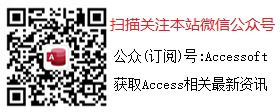# 自定义的从字符串中提取字母及数字的VBA函数

## 自定义的从字符串中提取字母及数字的VBA函数：

'提取所有字母及数字（包括全角及半角）：

Function ExtractAlphanumeric(strText As String) As String
Dim lngI As Long
Dim strChar As String
Dim strTemp As String
For lngI = 1 To Len(strText)
strChar = Mid\$(strText, lngI, 1)
If strChar Like "[A-z0-9]" Then
strTemp = strTemp & strChar
End If
Next
ExtractAlphanumeric = strTemp
End Function

'只提取半角字母及数字：
Function ExtractAsciiAlphanumeric(strText As String) As String
Dim lngI As Long
Dim strChar As String
Dim strTemp As String
For lngI = 1 To Len(strText)
strChar = Mid\$(strText, lngI, 1)
If (Asc(strChar) >= 65 And Asc(strChar) <= 90) or (Asc(strChar) >= 97 And Asc(strChar) <=

122) or (Asc(strChar) >= 48 And Asc(strChar) <= 57) Then
strTemp = strTemp & strChar
End If
Next
ExtractAsciiAlphanumeric = strTemp
End Function

Access软件网QQ交流群 (群号:32587638) 　　　　　　access源码网店

## 最新评论 查看更多评论(1)2019/8/12 10:49:03张俊： 收藏

## 发表评论您的评论将提升作者分享的动力！快来评论一下吧！

 用户名： 密　码： 内　容：

## 相关资源